# KVPY-SX 2017 Chemistry Paper with Solutions

KVPY-SX 2017 Chemistry paper with solutions is a perfect resource which helps the candidates in understanding their weaker areas. Candidates can download the previous years’ question papers of KVPY-SX to figure out the easiest and fastest way to answer a particular question. Practising the KVPY-SX previous year papers will guide the students to attempt each question with the best possible approach and helps in building time management skills. Solving these papers with solutions will help the students understand the concepts and prepare them to face the main exam with more confidence.

### KVPY SX 2017 - Chemistry

Question 1: The major product formed in the following reaction is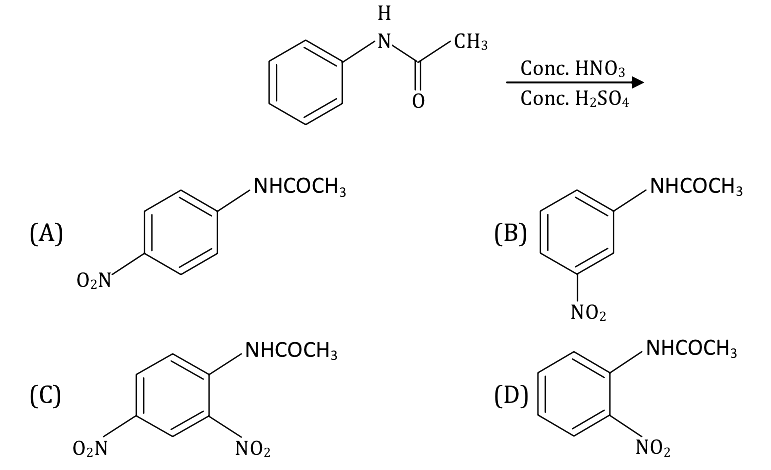Solution: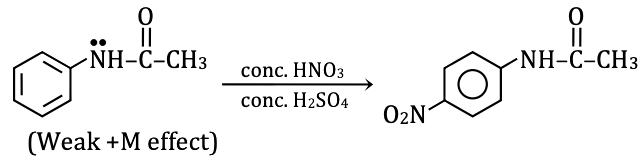In the above molecule, lone pair of nitrogen is in conjugation with benzene as well as a carbonyl group. Therefore, the +M effect of the ‘NH’ group on benzene will be weak. The only mono substitution will take place.

Question 2: Among the α-amino acids - threonine, tyrosine, methionine, arginine and tryptophan, those which contain an aromatic group in their side chain are

1. a. threonine and arginine
2. b. tyrosine and tryptophan
3. c. methionine and tyrosine
4. d. arginine and tryptophan

Solution: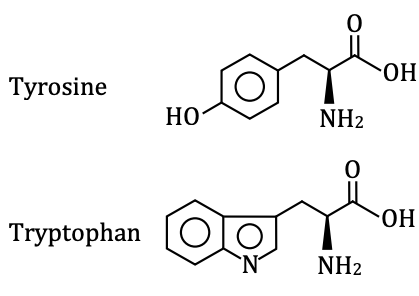Question 3: The number of stereoisomers possible for the following compound is CH3 – CH = CH – CH(OH) – CH3

1. a. 1
2. b. 2
3. c. 3
4. d. 4

Solution: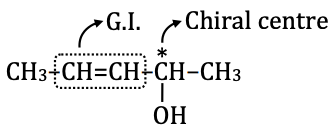n = 2

Number of possible stereoisomers = 2n = 22 = 4

G.I. → Can be cis/trans.

The chiral centre → can be R/S.

Therefore possible combination

Cis – R

Cis – S

Trans – R

Trans – S

Question 4: In electrophilic aromatic substitution reactions of chlorobenzene, the ortho/para directing ability of chlorine is due to its

1. a. Positive inductive effects (+I)
2. b. negative inductive effect (–I)
3. c. Positive resonance effect (+R)
4. d. negative resonance effect (–R)

Solution: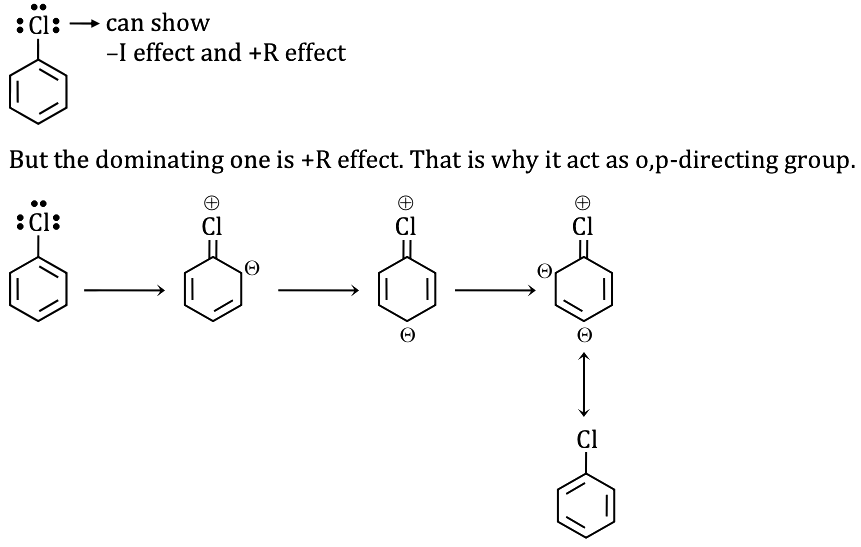Question 5: Among the following,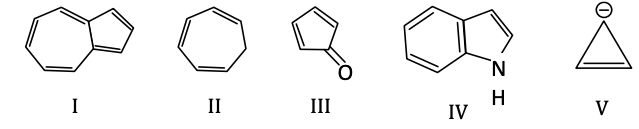the anti-aromatic compounds are

1. a. I and IV
2. b. III and V
3. c. II and V
4. d. I and III

Solution: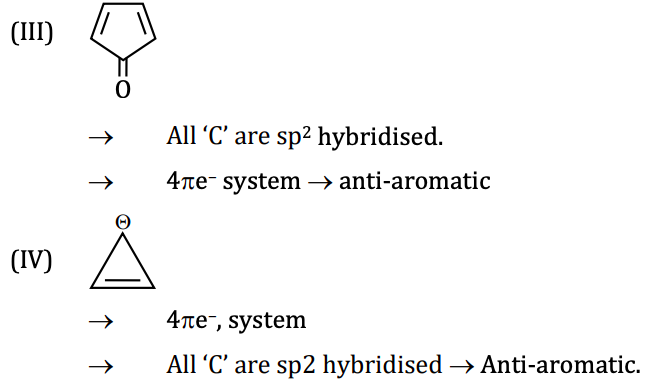Question 6: Upon reaction with CH3MgBr followed by protonation, the compound that produces ethanol is

1. a. CH3CHO
2. b. HCOOH
3. c. HCHO
4. d. (CHO)2

Solution: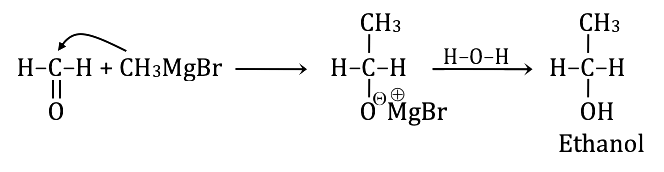Question 7: Which of the following is NOT an oxidation-reduction reaction?

1. a. H2 + Br2 → 2HBr
2. b. NaCl + AgNO3→NaNO3 + AgCl
3. c. 2Na2S2O3 + I2 → Na2S4O6 + 2NaI
4. d. Cl2 + H2O → HCl + HOCl

Solution: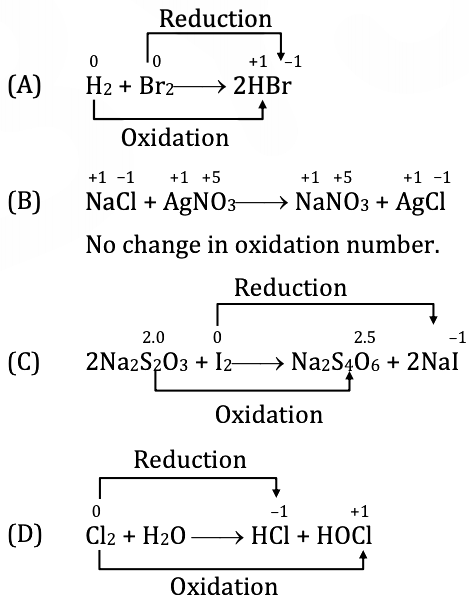Question 8: The thermal stability of alkaline earth metal carbonates – MgCO3, CaCO3, SrCO3 and BaCO3, follows the order

1. a. BaCO3 > SrCO3 > CaCO3 > MgCO3
2. b. CaCO3 > SrCO3 > BaCO3 > MgCO3
3. c. MgCO3 > CaCO3 > SrCO3 > BaCO3
4. d. SrCO3 > CaCO3 > MgCO3 > BaCO3

Solution:

Mg2+

Ca2+

Sr2+

Ba2+

Down the group, the size of cation increases and CO32– is also large in size.

And large cation + large anion → more stability.

Hence, more comparable the size of cation and anion, more will be the thermal stability of alkaline earth metal carbonates.

Hence, BaCO3 > SrCO3 > CaCO3 > MgCO3.

Question 9: When a mixture of diborane and ammonia is heated, the final product is

1. a. BH3
2. b. NH4BH4
3. c. NH2NH2
4. d. B3N3H6

Solution: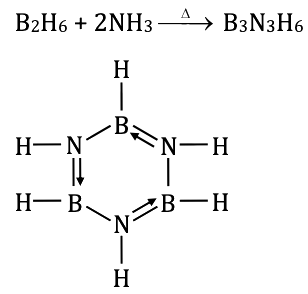Borazole or Inorganic benzene

Question 10: Among the following metals, the strongest reducing agent is

1. a. Ni
2. b. Cu
3. c. Zn
4. d. Fe

Solution:

According to electrochemical series,

 SRP Value Ni – 0.25V Zn – 0.76V Fe – 0.44V Cu + 0.34V

Lesser the value of E0RP, higher will be the tendency to get oxidized itself and reduce others and hence stronger will be the reducing agent.

Question 11: The molecule which is NOT hydrolysed by water at 25°C is

1. a. AlCl3
2. b. SiCl4
3. c. BF3
4. d. SF6

Solution:

a. AlCl3 + 3H2O → Al(OH)3 ↓ + 3HCl

(Al has vacant p-orbital where H2O can attack.)

b. SiCl4 + 4H2O → Si(OH)4 + 4HCl

(Si has vacant d-orbital where H2O can attack.)

c. BF3 + 3H2O → B(OH)3 + 3HF

(B has vacant p-orbital where H2O can attack.)

d. SF6 + H2O → (At 25o C) No reaction

Sulphur has vacant d-orbital but due to steric hindrance created by 6F, SF6 cannot be hydrolysed at 25°C.

Question 12: Among the following compounds, the one which does NOT produce nitrogen gas upon heating is

1. a. (NH4)2Cr2O7
2. b. NaN3
3. c. NH4NO2
4. d. (NH4)2(C2O4)

Solution:

(NH4)2Cr2O7 → N2 + Cr2O3 + H2O

2NaN3 → 2Na + 3N2

NH4NO2 → N2 + H2O

(NH4)2C2O4 → 2NH3 + H2C2O4

Question 13: Chlorine has two naturally occurring isotopes, 35Cl and 37Cl. If the atomic mass of Cl is 35.45, the ratio of the natural abundance of 35Cl and 37Cl is closest to

1. a. 3.5 : 1
2. b. 3 :1
3. c. 2.5 : 1
4. d. 4 : 1

Solution:

Atomic mass of Cl = 35.45 = Average atomic mass

Average atomic mass = [n1M1 + n2M2] / [n1 + n2]

35.45 = [n1 * 35 + n2 * 37] / [n1 + n2]

35.45n1 + 35.45n2 = 35n1 + 37n2

0.45n1 = 1.55n2

n1 / n2 = 1.55 / 0.45 ≈ 3 / 1

3 : 1

Question 14: The reaction C2H6(g) → C2H4(g) + H2(g) is at equilibrium in a closed vessel at 1000 K. The enthalpy change (ΔH) for the reaction is 137.0 kJ mol–l. Which one of the following actions would shift the equilibrium to the right?

1. a. Decreasing the volume of the closed reaction vessel
2. b. Decreasing the temperature at which the reaction is performed
3. c. Adding an inert gas to the closed reaction vessel
4. d. Increasing the volume of the closed reaction vessel

Solution:

C2H6(g) → C2H4(g) + H2(g); ΔH = +ve

a. Volume ↓ → Reaction will shift in the direction where the number of moles ↓ according to Le-chatelier principle → backward direction.

b. T ↓ → Reaction will shift in a direction where the temperature will increase (backwards) as the reaction is endothermic.

c. Addition of inert gas at constant volume (no effect on equilibrium).

d. Volume ↑ → Reaction will shift in a direction where the number of moles will increase i.e. forward direction.

Correct option is (d).

Question 15: The enthalpy (H) of an elementary exothermic reaction A -> B is schematically plotted against the reaction coordinate. The plots in the presence and absence of a catalyst are shown in dashed and solid lines, respectively. Identify the correct plot for the reaction.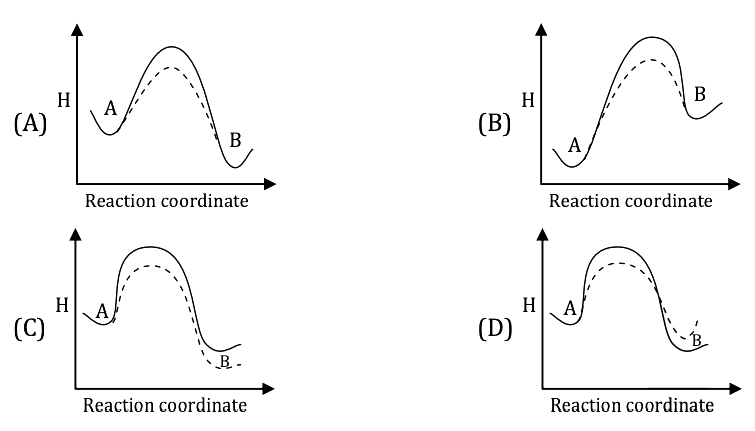Solution:

For exothermic reaction

ΔH = EP – ER < 0

EB – EA < 0

EB < EA

Catalyst does not change the initial and final position of the reaction. It will only decrease the activation energy, hence increase the rate of reaction.

Question 16: Mg(OH)2 is precipitated when NaOH is added to a solution of Mg2+. If the final concentration of Mg2+ is 10–10M, the concentration of OH(M) in the solution is [Solubility product for Mg (OH)2 = 5.6 × 10–12]

1. a. 0.056
2. b. 0.12
3. c. 0.24
4. d. 0.025

Solution:

Mg(OH)2(s) ⇌ Mg2+ + 2OH

Equilibrium s 2s

Ksp = [Mg2+][OH]2 = 5.6 × 10–12

10–10 [OH]2 = 5.6 × 10–12

[OH] = √5.6 × 10–2 = 0.24 M

Question 17: A constant current (0.5 amp) is passed for 1 hour through

(i) aqueous AgNO3,

(ii) aqueous CuSO4 and

(iii) molten AlF3, separately.

The ratio of the mass of the metals deposited on the cathode is [MAg, MCu, MAl are molar masses of the respective metals]

1. a. MAg: 2 MCu: 3 MA1
2. b. MAg: MCu: MA1
3. c. 6 MAg: 3 MCu: 2 MA1
4. d. 3 MAg: 2MCu: MA1

Solution: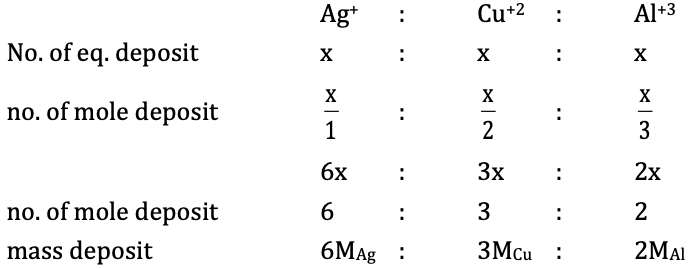Question 18: A reaction has an activation energy of 209 kJ mol–1. The rate increases 10–fold when the temperature is increased from 27°C to X °C. The temperature X is closest to [Gas constant, R = 8.314 J mol–1K–1]

1. a. 35
2. b. 40
3. c. 30
4. d. 45

Solution:

𝛆a = 209 kJmol–1

Given:

T1 = 27°C = 300 K

T2 = x°C = (x + 273)K

K2 / K1 = 10

Apply

log (K2 / K1) = [𝛆a / 2.303 R] [(1 / T1) - (1 / T2)]

1 = log 10 = {[209 * 103] / [2.303 * 8.314]} {[(1 / 300) - (1 / (x + 273)]}

x + 273 = 308.4 K

x = 35.4°C ≈ 35°C

Question 19: A mineral consists of a cubic close-packed structure formed by O2–ions, where half the octahedral voids are occupied by Al3+ and one-eighth of the tetrahedral voids, are occupied by Mn2+. The chemical formula of the mineral is

1. a. Mn3Al2O6
2. b. MnAl2O4
3. c. MnAl4O7
4. d. Mn2Al2O5

Solution:

No. of atoms in ccp = N

Then, No. of O.V. = N

No. of T.V. = 2N

No. of O–2 per unit cell = 8 × (1 / 8) + 6 × (1 / 2) = 4

No. of Al+3 per unit cell = 4 × (1 / 2) = 2

No. of Mn+2 per unit cell = 8 × (1 / 8) = 1

MnAl2O4

Question 20: For a 4p orbital, the numbers of radial and angular nodes, respectively, are

1. a. 3, 2
2. b. 1, 2
3. c. 2, 4
4. d. 2, 1

Solution:

4p

n = 4, l = 1

No. of Radial nodes = n – l – 1 = 4 – 1 – 1 = 2

No. of Angular nodes = l = 1

Question 21: In the following reaction sequence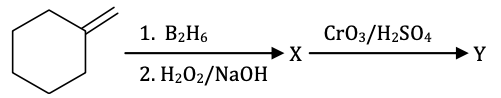X and Y are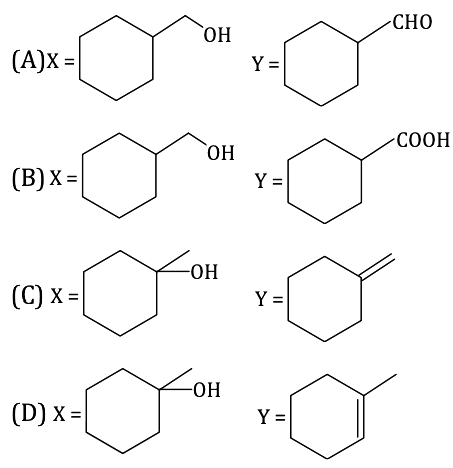Solution: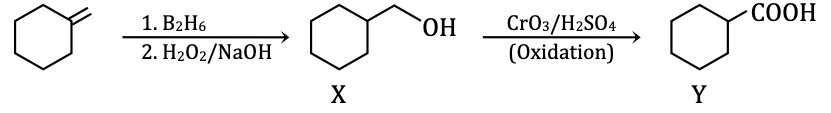[Hydroboration-Oxidation Reaction]

Anti-markovnikov’s rule

Question 22: In the following reactions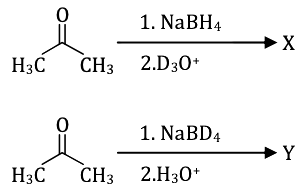X and Y are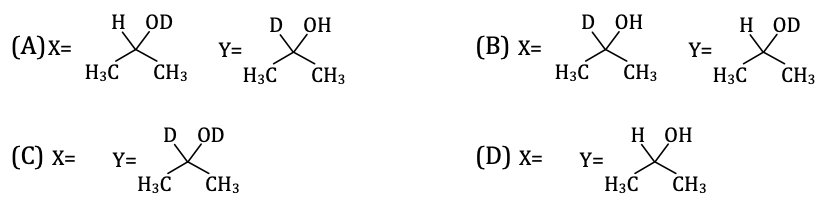Solution: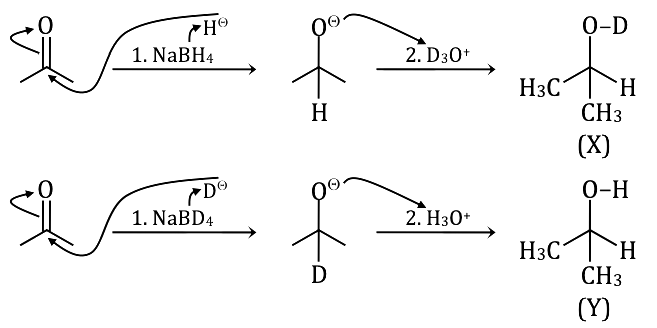Question 23: Which of the following alkenes can generate optically active compounds upon hydrogenation?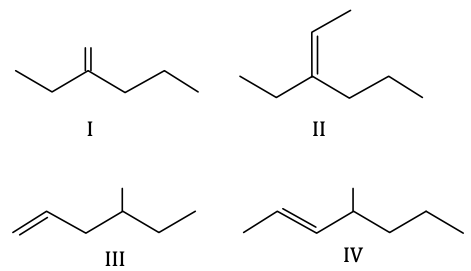1. a. I, III and IV
2. b. II and III
3. c. I and III
4. d. II and IV

Solution: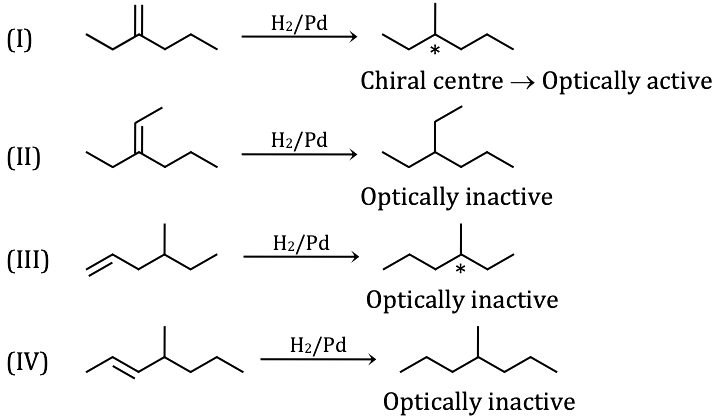Question 24: When heated in air, brown copper powder turns black. This black powder would turn brown again when heated with

1. a. CO b. O2c. H2d. NH3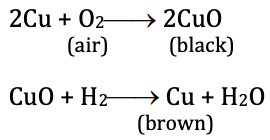Solution:Question 25: The geometry and magnetic property of [NiCl4]2–, respectively, are

1. a. Tetrahedral, paramagnetic
2. b. tetrahedral, diamagnetic
3. c. Square planar, paramagnetic
4. d. square planar, diamagnetic

Solution: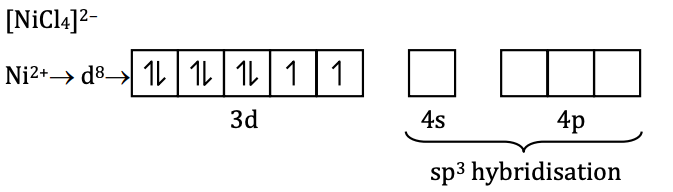Cl is a weak ligand

No pairing of the electron will take place.

• sp3 hybridisation, tetrahedral

• 2 unpaired electrons → paramagnetic

Question 26: Among

(i) [Cr(en)3]3+,

(ii) trans-[Cr(en)2Cl2]+,

(iii) Cis-[Cr(en)2Cl2]+

(iv) [Co(NH3)4Cl2]+

the optically active complexes are

1. a. i and ii
2. b. i and iii
3. c. ii and iii
4. d. ii and iv

Solution: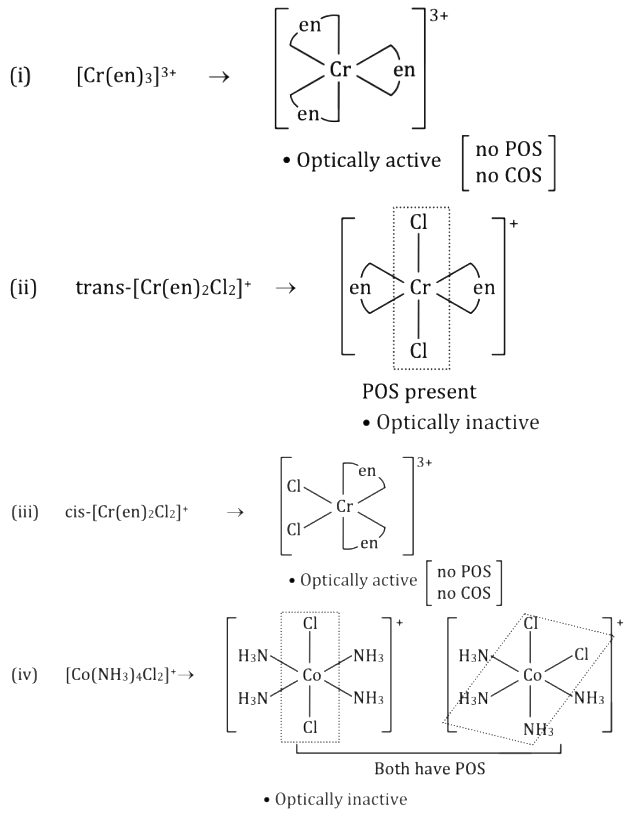Question 27: 227Ac has a half-life of 22 years with respect to radioactive decay. The decay follows two parallel paths: 227Ac → 227Th and 227Ac → 223Fr. If the percentage of the two daughter nuclides are 2.0 and 98.0, respectively, the decay constant (in year–1) for 227Ac → 227Th path is closest to

1. a. 6.3 × 10–2
2. b. 6.3 × 10–3
3. c. 6.3 × 10–1
4. d. 6.3 × 10–4

Solution: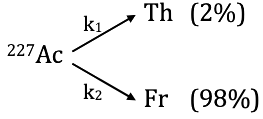k1 / k2 = 2 / 98

t½ = 22 years

k = 0.693 / t½ = 0.693 / 22 year = k1 + k2

0.693 / 22 = k1 + (98 / 2) k1

k1 = 6.3 × 10–4

Question 28: A system consisting of 1 mol of an ideal gas undergoes a reversible process, A → B → C → A (schematically indicated in the figure below). If the temperature at the starting point A is 300 K and the work done in the process B → C is 1 L atm, the heat exchanged in the entire process in L atm is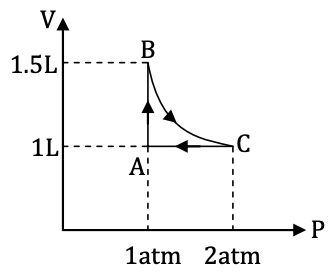1. a. 1.0
2. b. 0.0
3. c. 1.5
4. d. 0.5

Solution:Given: n = 1 mole, TA = 300 K, ωBC = 1 L-atm

ωAB = –Pext.(V2 – V1) = –1(1.5 – 1) = –0.5 L-atm

(Isobaric process)

ωCA = 0 (dV = 0)

(Isochoric process)

ωtotal = ωAB + ωBC + ωCA

= –0.5 + 1 + 0 = 0.5 L-atm

ΔU calculation

For complete cyclic process ABC → ΔU = 0 (As U → is a state function) According to first law,

ΔU = q + w = 0

q = –w = –0.5 L-atm

Heat exchanged during the process = 0.5 L-atm.

Correct option is (D).

Question 29: A mixture of toluene and benzene boils at 100°C. Assuming ideal behaviour, the mole fraction of toluene in the mixture is closest to [Vapour pressures of pure toluene and pure benzene at 100°C are 0.742 and 1.800 bar respectively. 1 atm = 1.013 bar]

1. a. 0.824
2. b. 0.744
3. c. 0.544
4. d. 0.624

Solution:

toluene = 0.742 bar

benzene = 1.800 bar

Mixture boils at 100°C → at 1 atm = 1.013 bar

PT = 1.013 bar = PAXA + PBXB

PT = Pot Xt + Pob Xb

1.013 = 0.742 Xt + 1.8 (1 – Xt)

Xt = 0.744

Xb = 1 – 0.744 = 0.256

Xt = mole fraction of toluene.

Question 30: A two-dimensional solid pattern formed by two different atoms X and Y is shown below. The black and white squares represent atoms X and Y, respectively. The simplest formula for the compound based on the unit cell from the pattern is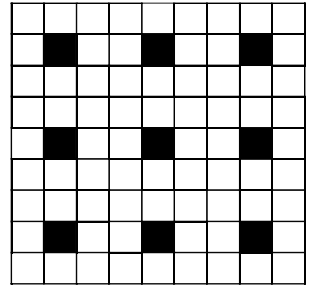1. a. XY8
2. b. X4Y9
3. c. XY2
4. d. XY4

Solution:

The unit cell of the above pattern will consist of 8 white squares and 1 black square i.e. it will form the centre unit cell.

No. of white square Y = 8

No. of black square X = 1

Formula XY8.

### KVPY SX 2017 Chemistry Paper with Solutions